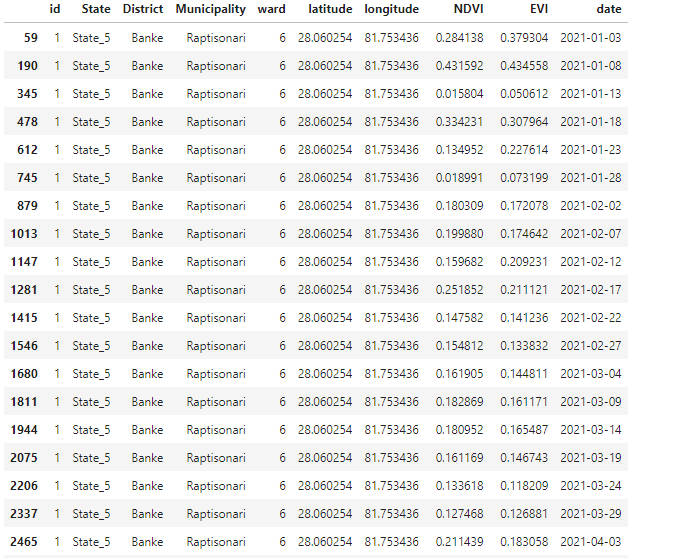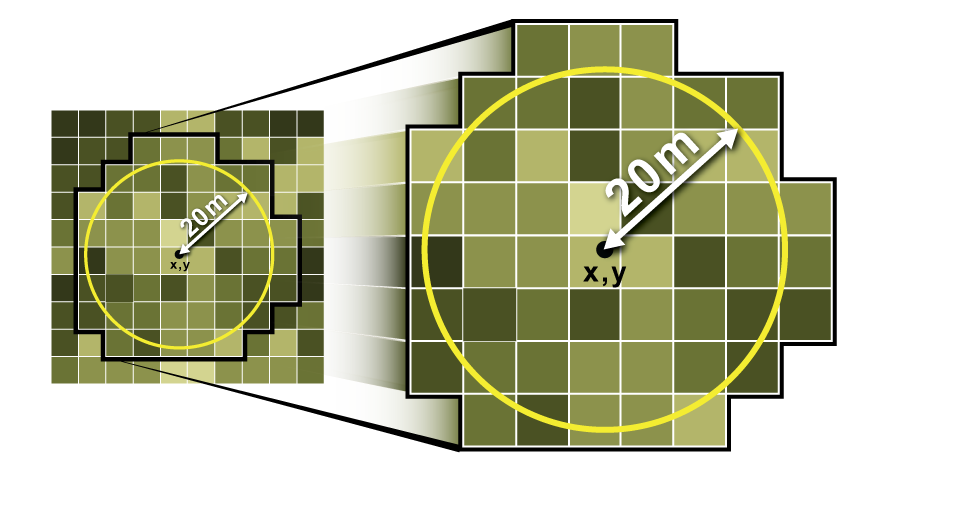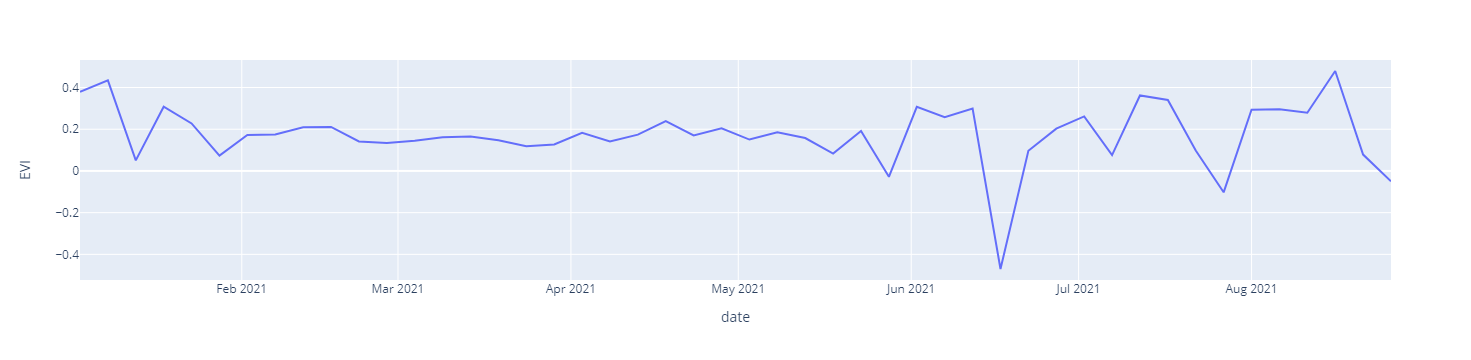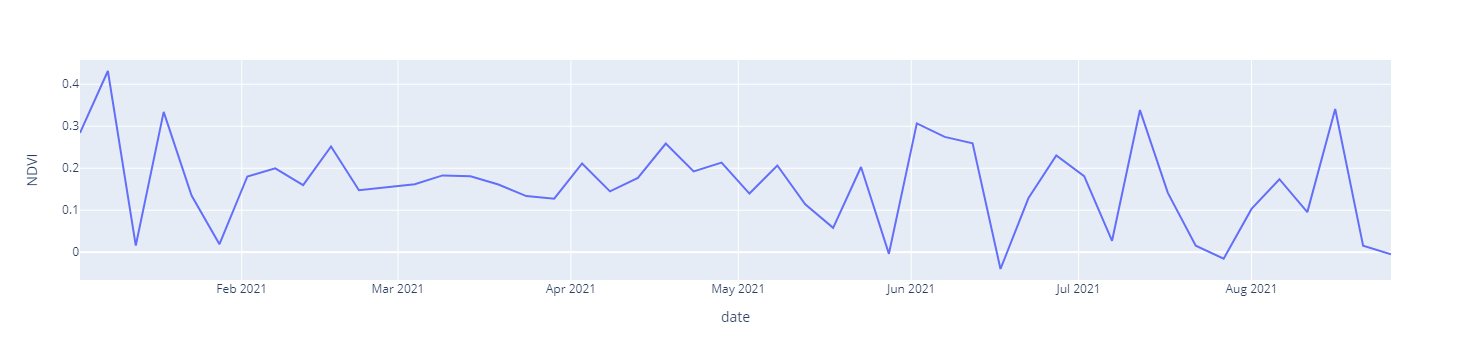# Extraction of raster values from point samples on Google Earth Engine (GEE)## Background:

In this tutorial, We plan to extract the raster cell values from the set of point features nd records the values in the attribute table as an output. on Google Earth Engine (GEE) with python API. Before we dive into the working steps, I will explain the general idea of how this concept can be implemented.
The process of raster value extraction can be implemented in two ways,

• Directly extracting the raster values by overlaying raster with point data. Raster value which perfectly overlays with the point is taken.
• Buffer (circle, square) around the point is created, and raster statistics (mean) are obtained. This mean is taken as the raster value for points. Refer this
•## Scenario, where you are going to need raster value extraction:

At some point, anyone working with field data collected in plots will almost certainly need to extract raster data for those plots. For example, the Normalized Difference Vegetation Index (NDVI) is a widely used measure of vegetation greenness that can be derived using a number of satellite datasets. You might also want to plot climate data or extract reflectance from multispectral satellite bands to compute your own indices later. This method can be used on a single image or a collection of images. When you run the procedure across an image collection, you'll get a table with values from each image per point. Before extraction, Image collections can be treated as appropriate, such as masking clouds from satellite imagery.

Now Let's move into the steps, where the concept is implemented.

### Implementation of raster value extraction from points on Google Earth Engine (GEE):

1. Importing modules
``````# import Google earth engine module
import ee

# First firt setup only, no need to run this after first run

# ee.Authenticate()

# for normal/regular use for authorization
# this is required regularly
ee.Initialize()

#Pandas modules to interact data
import numpy as np
import pandas as pd``````
2. Define indicators of your interest. For this blog, i will demonstrate NDVI and EVI for Sentinel-2 image

``````# compute NDVI from NIR and red band in sentinel -2 image
# For other satellite image, please change the band information accordingly

def getNDVI(image):

# Normalized difference vegetation index (NDVI)
ndvi = image.normalizedDifference(['B8','B4']).rename("NDVI")

return(image)

# compute EVI from NIR and red band in sentinel -2 image
# For other satellite image, please change the band information accordingly

def getEVI(image):
# Compute the EVI using an expression.
EVI = image.expression(
'2.5 * ((NIR - RED) / (NIR + 6 * RED - 7.5 * BLUE + 1))', {
'NIR': image.select('B8').divide(10000),
'RED': image.select('B4').divide(10000),
'BLUE': image.select('B2').divide(10000)
}).rename("EVI")

return(image)``````
3. Date parameter for image
It is most important part for time series study.
``````# manage the date formating as per your requirements
# Mine is in format of YYYYMMdd
img_date = ee.Date(image.date())
img_date = ee.Number.parse(img_date.format('YYYYMMdd'))
4. Filter the imagery for your desired timeframe and other parameters
I have limited the filters for sample only.
``````Sentinel_data = ee.ImageCollection('COPERNICUS/S2') \
.filterDate("2021-01-01","2021-08-31") \
5. Import your point samples here.
``````plot_df = pd.read_excel('data/sample_plot_points_random.xlsx')
plot_df``````
6. Convert the pandas dataframe into Google Earth Engine (GEE) Feature Collection
``````features=[]
for index, row in plot_df.iterrows():
#     print(dict(row))
#     construct the geometry from dataframe
poi_geometry = ee.Geometry.Point([row['longitude'], row['latitude']])
#     print(poi_geometry)
#     construct the attributes (properties) for each point
poi_properties = dict(row)
#     construct feature combining geometry and properties
poi_feature = ee.Feature(poi_geometry, poi_properties)
#     print(poi_feature)
features.append(poi_feature)

# final Feature collection assembly
ee_fc = ee.FeatureCollection(features)
ee_fc.getInfo()``````
7. Extract the raster values for each features
We will use sampleRegions function from ee.image and apply to image collection
``````def rasterExtraction(image):
feature = image.sampleRegions(
collection = ee_fc, # feature collection here
scale = 10 # Cell size of raster
)
return feature
``````
8. Apply raster extraction functions over image collection
sampleRegions returns feature collection with image values, then we have collection of feature collection which is then flattened to obtain final feature collection. Finally feature collection is converted to CSV.
``results = Sentinel_data.filterBounds(ee_fc).select('NDVI', 'EVI').map(addDate).map(rasterExtraction).flatten()``
9. Verify that output is as per your requirments
``````sample_result = results.first().getInfo()
sample_result``````
10. Now we have extracted the raster values, We need to convert feature collection to CSV format.
We can acheive this in multiple ways, I will illustrate in 3 ways for extraction.
``````# extract the properties column from feature collection
# column order may not be as our sample data order
columns = list(sample_result['properties'].keys())
print(columns)

# Order data column as per sample data
# You can modify this for better optimization
column_df = list(plot_df.columns)
column_df.extend(['NDVI', 'EVI', 'date'])
print(column_df)

``````
11. ## Method 1: Feature collection to pandas dataframe

``````nested_list = results.reduceColumns(ee.Reducer.toList(len(column_df)), column_df).values().get(0)
data = nested_list.getInfo()
data

# dont forget we need to call the callback method "getInfo" to retrieve the data
df = pd.DataFrame(data, columns=column_df)
# we obtain the data frame as per our demand
df``````

``````url_csv = results.getDownloadURL('csv')
# You can use this url and download with python request module, I will leave that to you
url_csv
``````

``````task = ee.batch.Export.table.toDrive(**{
'collection': results,
'description':'vectorsToDriveExample',
'fileFormat': 'csv'
})

import time
time.sleep(5)``````
14. Data visualization of results:
I will go on details for visualization on the next blog post.
``````# import libraries

# Using plotly.express
import plotly.express as px

# I will plot line plot for single point only for now.
# I will cover detail analaysis in next plot
df_filtered = df[df['id']==1]
df_filtered['date'] = pd.to_datetime(df_filtered['date'], format='%Y%m%d')
df_filtered``````
15. ``````df = px.data.stocks()
fig = px.line(df_filtered, x='date', y="EVI")
fig.show()

df = px.data.stocks()
fig = px.line(df_filtered, x='date', y="NDVI")
fig.show()``````

Here's some sample for the visualized data. This chart data are highly affected by the cloud cover as I have taken all sentinel -2 data without any cloud mask.EVI vs DateNDVI vs Date

I will be bringing next post on details for the time series analysis of various vegetation indices.

Please let us know your thoughts on this with following comment section we will definetly reach out to you on your query and feedback.

##### Gedefaye Achamu | General comments

Oct. 8, 2022, 5:37 p.m.

Thanks for your contribution I think you would not mind if I used it for my case Suggestion Would you have something toue regarding com say on an computation time to methods one and two? The first fails to compute while the latter takes a while Thank you

##### kampus jatim | https://www.um-surabaya.ac.id/

May 27, 2023, 3:50 a.m.

Thanks for sharing this useful information! Hope that you will continue with the kind of stuff you are doing.